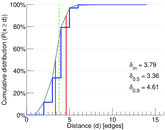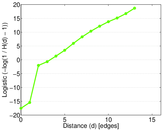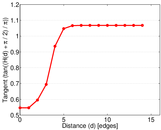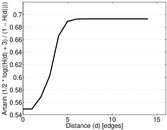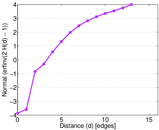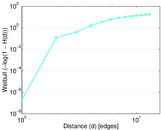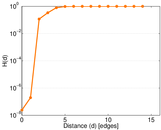# Web trackers

This is the bipartite network of internet domains and the trackers they contain, such as the Facebook button and Google Analytics. Left nodes are domains and right nodes are trackers, identified also by their domain.

 Code `WT` Internal name `trackers-trackers` Name Web trackers Data source https://ssc.io/trackingthetrackers/ AvailabilityDataset is available for download Consistency checkDataset passed all tests Category Hyperlink network Dataset timestamp 2012 Node meaning Domain, tracker Edge meaning Inclusion Network formatBipartite, undirected Edge typeUnweighted, no multiple edges

## Statistics

 Size n = 40,421,974 Left size n1 = 27,665,730 Right size n2 = 12,756,244 Volume m = 140,613,762 Wedge count s = 106,441,673,202,003 Claw count z = 2.963 5 × 1020 Cross count x = 7.817 07 × 1026 Maximum degree dmax = 11,571,952 Maximum left degree d1max = 1,100,065 Maximum right degree d2max = 11,571,952 Average degree d = 6.957 29 Average left degree d1 = 5.082 60 Average right degree d2 = 11.023 1 Fill p = 3.984 40 × 10−7 Size of LCC N = 39,821,755 Diameter δ = 21 50-Percentile effective diameter δ0.5 = 3.359 14 90-Percentile effective diameter δ0.9 = 4.608 99 Median distance δM = 4 Mean distance δm = 3.789 13 Gini coefficient G = 0.734 715 Balanced inequality ratio P = 0.219 572 Left balanced inequality ratio P1 = 0.274 646 Right balanced inequality ratio P2 = 0.119 211 Relative edge distribution entropy Her = 0.762 650 Power law exponent γ = 2.212 82 Tail power law exponent γt = 2.161 00 Degree assortativity ρ = −0.057 485 9 Degree assortativity p-value pρ = 0.000 00 Spectral norm α = 3,790.86

## Plots

### Degree distribution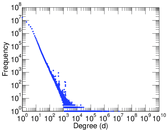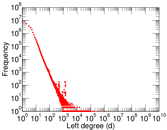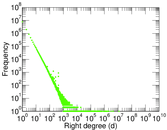### Cumulative degree distribution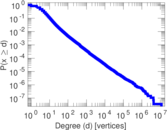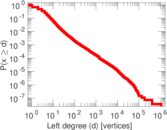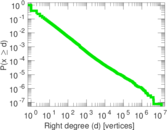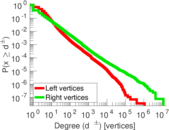### Lorenz curve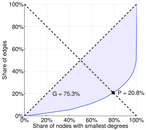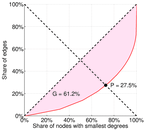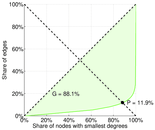### Spectral distribution of the adjacency matrix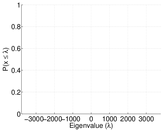### Spectral distribution of the normalized adjacency matrix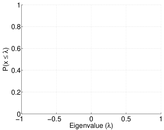### Spectral distribution of the Laplacian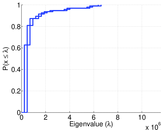### Spectral graph drawing based on the adjacency matrix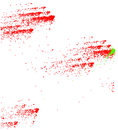### Spectral graph drawing based on the normalized adjacency matrix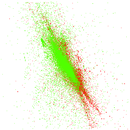### Hop distribution